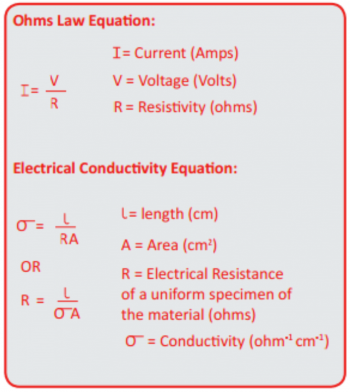Everything Eddy Current

Resources

# Introduction to Conductivity

Please find following a basic introduction to conductivity and Eddy Current (ECT) Conductivity Measuring within Non-destructive Testing (NDT).

Electrical conductivity is the measurement of a materials ability to conduct an electric current. This is the inverse of electrical resistivity, measuring a materials ability to resist an electric current.

Conductivity in metal is established using Ohm’s Law, which states that current through a conductor, between two points, is directly proportional to the potential difference across the two points. The resistance of the material, which is a constant for that material, allows the usual mathematical equation for this relationship to be true.

Conductivity is widely used to indicate material type and determine the state of heat treatment. In order to give accurate readings the SigmaCheck uses a three-point reference method. The first measurement with the probe in the air and then two further measurements are required which span the range of interest.### Measuring Conductivity with the SigmaCheck

The SigmaCheck 2 is supplied with a detachable reference piece with two standards that span the range of commonly used metals.

ETher NDE also manufacture individual Conductivity Test Blocks which may be used to match the clients own testing requirements. We can also provide a handy test block holder that can house up to five of these test blocks at any one time.

Resources